Courses
Courses for Kids
Free study material
Offline Centres
More

# Laws of Motion Chapter - Physics JEE MainLast updated date: 02nd Dec 2023
Total views: 21.9k
Views today: 0.21k## Concepts of Laws of Motion for JEE Main Physics

The chapter on laws of motion starts with the basic revision of all the concepts we studied in our lower grades to build the connection between the advanced parts of the laws of motion. In laws of motion, we have discussed Newton's laws of motion, Inertia, derivation of the second law of motion, conservation law, etc….

While deriving the second law of motion we study the relation between the force and momentum, $\vec {F}=\dfrac {d \vec {P}}{dt}$. This relation further leads us to study the conservation of linear momentum which helps us in solving most of the problems related to motion. In the chapter laws of motion, we also studied the force and types of forces along with examples.

We got introduced to a few most important concepts: friction and laws of friction along with the concept of tension. We studied how to determine the forces acting on the body with the help of free body diagrams.

In this chapter students also get to learn about the circular motion where we study an object in circular motion experiencing the different forces (i.e., Centrifugal and Centripetal force). Students will also study the “frames of reference”, a very interesting concept of classical mechanics.

Now, let us move on to the important concepts and formulae related to JEE and JEE main exams along with a few solved examples.

## JEE Main Physics Chapters 2024

### Important Topics of Laws of Motion Chapter

• Newton’s Law of Motion Formula

• Linear Momentum

• Conservation of Linear Momentum

• Types of Forces

• Friction and types

• Laws of Friction

• Resultant force

• Tension

• Simple pulley system

### Laws of Motion Important Concept for JEE Main

 Name of the Concept Key Points of Concept 1. Newton's Law of Motion Newton's First Law of MotionNewton's First Law of Motion, also known as the law of inertia, states that an object at rest will stay at rest, and an object in motion will stay in motion with the same speed and in the same direction unless acted upon by an unbalanced external force. The key points to remember are:Inertia: Every object has a natural tendency to resist changes in its state of motion.No net force = No acceleration.Newton's Second Law of MotionNewton's Second Law of Motion relates the net force acting on an object to its acceleration and mass. Mathematically, it can be expressed as F = ma, where:F is the net force applied to an object.m is the mass of the object.a is the acceleration produced by the force.This law provides a quantitative relationship between force, mass, and accelerationNewton's Third Law of MotionNewton's Third Law of Motion states that for every action, there is an equal and opposite reaction. It means that when one object exerts a force on a second object, the second object exerts an equal and opposite force on the first. This law explains how interaction between objects occurs. 2. Linear Momentum and Impulse Linear Momentum is defined as the product of mass and linear velocity, $\vec{P}=m v$Impulse force acting on a body is defined as the product of force and the infinitesimally small interval of time for which it acts,$I=\int_{t_1}^{t_2}\vec {F} dt=\vec {P_2}-\vec{P_1}$ 3. Conservation of Linear Momentum The law of conservation of linear momentum states that “when external forces are not acting, then total momentum of the body remains constant.” In other words, the momentum of the body before impact will be the same as the after impact. Mathematically we write:${m_1}{v_1}={m_2}{v_2}\Rightarrow {v_1}=\dfrac {{m_2}{v_2}}{m_1}$ 4. Concurrent Forces and Equilibrium When a group of forces will be acting on a single point, such forces are known as the concurrent forcesIf the resultant force of these forces is zero, then these concurrent forces are said to be in “equilibrium”. 5. Resultant Force Resultant force is the vector sum of all the forces acting on the body. 6. Friction Friction is the opposition offered when any object is moving a plane.The formula of the friction force is:$\vec {F}=\mu N$Relation between the coefficient of friction and of friction $\theta$:$\theta=\tan^{-1}\mu$ 7. Laws of Friction The total magnitude of limiting frictional force is always proportional to the normal force acting at the contact surface.The magnitude of limiting frictional force is always independent of the total surface area of contact between the surfaces. 8. Tension and Pulley System Tension is defined as a force acting on a stretched string or body. A pulley system is a basic physical apparatus with two or more masses attached to it. 9. Calculation of Tension Acting On a Body The direction of the tension acting on the string is always considered along the string and in a direction opposite to the weight of the body under consideration.$\Rightarrow T=m g$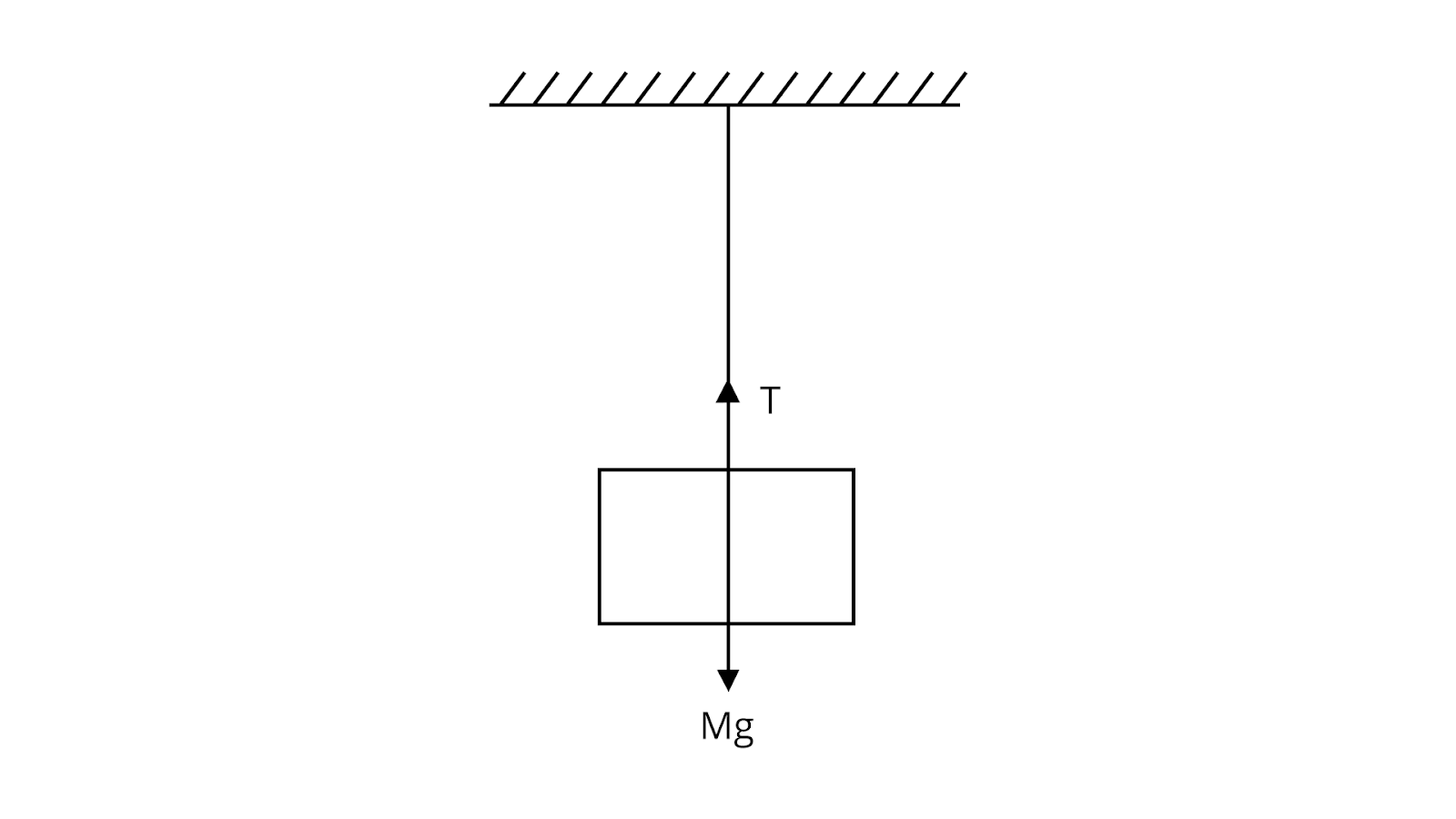A block Attached to a String 10. Calculation of Tension In a Simple Pulley The common acceleration ‘a’ acting in a simple pulley system:$a=\dfrac {{m_1}-{m_2}}{{m_1}+{m_2}} g$The tension in the string is given by$T=\dfrac {2{m_1}{m_2}}{{m_1}+{m_2}} g$Where, m1>m2

## Examples of Newton’s Law of Motion in Daily Life

### Newton’s First Law of Motion:

• A small coin is put on a card and placed over a glass. When the card is flicked away with the finger, the coin drops into the glass.

• Suppose we are sitting in a stationary bus. If it starts moving suddenly, we will feel a jerk in the backward direction. It is because our lower body is in contact with the seat of the bus that comes in motion as the bus starts moving, while the upper portion of our body remains at the rest due to inertia, and so we feel a jerk in the backward direction.

• Similarly, if we push a ball on the ground, it should continue its uniform motion indefinitely, but it stops after covering a certain distance. As soon as the ball starts moving, a force (force of friction) comes into play, which opposes the motion of the ball.

• A book lying on a table can't change its position by itself unless a force is applied to change its position.

### Newton’s Second Law of Motion:

• Pushing or pulling an empty cart is easy as compared to a loaded cart because the loaded cart has more mass.

• If the same amount of force is applied to move a car and a bike, the acceleration of the bike will be more because it has less mass than the car.

• When a ball drops on the ground, it exerts a downward force on the ground, and in reaction to it the ground exerts an equal upward force on the ball, thus making it bounce.

• Stopping a moving ball requires force.

### Newton’s Third Law of Motion:

• When a man or woman is out on a stroll, he/she exerts force in a backward direction on the ground. On the other hand, the ground puts a forward directional force on the man/woman that makes him/her move forward.

• Another Newton's 3rd law example is when an individual is swimming, he/she pushes the pool water backward. In return, the water pushes the swimmer in a forward direction, which makes him/her proceed.

• Agreeing with Newton's third law, a bird pushes air downwards with the help of its wings. Conversely, the air helps the bird to move in the upward direction.

## Derivation of First Equation of Motion

Let’s Consider a body of mass m having initial velocity u.

Let after time be t its final velocity becomes v due to uniform acceleration a.

Now it is defined as:

Acceleration = Change in velocity / Time taken

Acceleration = (Final velocity - Initial velocity) / Time taken

$a = \dfrac{(v - u)}{t}$

$a t = v - u$

or  $v = u + at$

This describes the first equation of motion.

## Derivation of Second Equation of Motion

As it is defined, the Second equation of motion: $s = ut + \dfrac{1}{2}at^2$

Let’s take the distance travelled by the body be s.

Now:

Distance = Average velocity x Time

Also, Average velocity $= \dfrac{(u + v)}{2}$

Therefore, Distance $(t) = \dfrac{(u + v)}{2t}$       ......eq.(1)

Again from first equation of motion:

$v = u + at$

Substituting this value of v in eq.(1), we get

$s = \dfrac{(u + u + at)}{2t}$

$s = \dfrac{(2u + at)}{2t}$

$s = \dfrac{(2ut + at^2) }{2}$

$s = \dfrac{2ut}{2} + \dfrac{at^2}{2}$

or  $s = ut + \dfrac{1}{2} at^2$

This describes the second equation of motion.

### Derivation of Third Equation of Motion

As it is defined that the third equation of Motion:  v2 = u2 + 2as

Now,

v = u + at

v - u = at

or  $t = \dfrac{(v - u)}{a}$            ........ eq.(2)

Also ,

Distance = average velocity x Time

Therefore, $s = \dfrac{v + u}{2} \times \dfrac{v - u}{a}$

$s = \dfrac{(v^2 - u^2)}{2a}$

$2as = v^2 - u^2$

or  $v^2 = u^2 + 2as$

This describes the third equation of motion.

### Inertia:

Inertia is the property of a body due to which it opposes any change in its state. The mass of a body is the measure of its inertia of translational motion. It is difficult to change the state of rest or uniform motion of a body of heavier mass and vice-versa. In simple words, inertia is a measure of the tendency of an object at rest to stay at rest or of an object in motion to stay in motion.

• Mass of a body is a quantitative or numerical measure of a body's inertia.

• Larger the inertia of a body, the more will be its mass.

### The Inertia of Rest

A body cannot change its state of rest by itself.

Examples:

• When we shake a branch of a mango tree, the mangoes fall.

• When a bus or train starts suddenly, the passengers sitting inside tend to fall backward.

• When a horse starts suddenly, the rider falls backwards.

• The dust particles in a blanket fall off when it is beaten with a stick.

• A coin is placed on cardboard, and this cardboard is placed over a tumbler, such that the coin is above the mouth of the tumbler. Now, if the cardboard is removed with a sudden jerk, the coin falls into the tumbler.

### The Inertia of Motion

A body cannot change its state of uniform motion by itself.

Examples:

• When a bus or train stops suddenly, the passengers sitting lean forward.

• A person who jumps out of a moving train may fall in the forward direction.

• A bowler runs the ball before throwing it so that this speed of running gets added to the speed of the ball at the time of the throw.

• An athlete runs through a certain distance before taking a long jump because the velocity acquired during the running gets added to the velocity of an athlete at the time of the jump, and hence he can jump over a longer distance.

• A ball is thrown in the upward direction by a passenger sitting inside a moving train.

The ball will fall:-

• back to the hands of the passenger, if the train is moving with constant velocity.

• ahead of the passenger, if the train is retarding (slowing down).

• behind the passenger, if the train is accelerating (speeding up).

### The Inertia of Direction

A body cannot change its direction of motion by itself. The tendency of an object to oppose any change (unbalanced force) in its direction of motion and continue to stay in direction is called inertia of direction.

Examples:

• When a straight running car turns sharply, the person sitting inside feels a force radially outwards.

• Rotating wheels of vehicles throw out mud, mudguards fitted over the wheels prevent this mud from spreading.

• When a knife is pressed against a grinding stone, the sparks produced move in the tangential direction.

## What is an External Force?

According to Newton's first law of motion, a push or a pull that either changes or tends to change the state of rest or uniform motion (constant velocity) of a body is known as a force. An external force is a force originating from outside an object rather than a force internal to an object.

For instance, the force of gravity that Earth exerts on the moon is an external force on the moon. However, the force of gravity that the inner core of the moon exerts on the outer crust of the moon is an internal force on the moon. Internal forces within an object can't cause a change in that object's overall motion.

### Effects of Resultant Force

• It may change the speed of the body.

• It may change the direction of motion.

• It may change both the speed and direction of motion.

•  It may change the size or/and the shape of the body.

• It may start a motion in a stationary body or it may stop a moving body.

### Units for Measurement of Force

Absolute units are-

• N (M.K.S)

• dyne (C.G.S)

Other units are-

• kg-wt or kg-f {kf-force}

• g-wt or g-f

## Acceleration and Velocity

Newton's second law of motion describes that, when a force is applied to an object, it produces acceleration in the object (i.e rate of change of velocity). For an object at rest, the applied force produces acceleration in the object and makes the object move in the direction of applied force.

For an object which is already in motion, the direction of the applied force matters to determine its state. If external force is applied in the direction in which the object is moving, the acceleration of the object increases. If external force is applied in the direction opposite to the motion of the object, the acceleration of the object decreases and finally comes to stop.

Force and acceleration are vector quantities, i.e they have both magnitude and direction. Multiple forces can also act in a body at a time.

Hence,

$\Sigma$F = ma

Where;

$\Sigma$= vector sum of all the forces acting on a body (net force).

### For Changing the Mass

For this let’s assume that we have a car at a point (0) which is defined by the location X0 and time t0. The car has a mass of m0 and travels with a velocity of v0. Here, after being subjected to a force ‘F’, the car starts to move to point 1, defined by location X1 and the time by t1. The mass and the velocity of the car changes during the travel to values m1 and v1 respectively. Thus, Newton's second law helps to determine the new values of m1 and v1 if we already know the value of the acting force.

From the difference of point 1 and point 0, an equation for the force acting on the car is formed as follows:

F=m1v1−m0v0t1−t0

F=m1v1−m0v0t1−t0

Now, let’s assume the mass to be constant here. This assumption is helpful for a car as the only change in the mass would be the fuel burned while moving between point “1” and point “0”. Here, the weight of the fuel is probably very small as compared to the rest of the car, especially looking at the small changes in time. Meanwhile, if we discuss the flight of a bottle rocket, then the mass does not remain constant here, and only the changes in momentum can be looked at.

### For Constant Mass

For a constant mass, Newton’s second law can be equated as follows:

F=mv1−v0t1−t0

F=mv1−v0t1−t0

We know, acceleration is defined as the change in velocity which is divided by the change in time.

The second law then decreases to a more common form as follows:

F=ma

The above equation conveys to us that an object will accelerate if it is subjected to an external force. While the amount of force is directly proportional to the acceleration and inversely proportional to the object’s mass.

### Law of Conservation of Linear Momentum:

The law of conservation of linear momentum states that the total linear momentum of a system of objects remains constant unless an external force acts on the system. Linear momentum is defined as the product of mass and velocity (p = mv).

The law of conservation of linear momentum can be applied to a variety of problems, including:

• Collisions between objects

• Explosions

• Rocket motion

• Motion of objects in a gravitational field

### Applications of the Law of Conservation of Linear Momentum:

• Collisions: When two objects collide, the total linear momentum of the system before the collision is equal to the total linear momentum of the system after the collision. This principle is used in the design of airbags and other safety devices.

• Explosions: When an object explodes, the total linear momentum of the system before the explosion is equal to the total linear momentum of the system after the explosion. This principle is used in the design of explosives and rockets.

• Rocket motion: Rockets work by expelling mass at high speed. The law of conservation of linear momentum tells us that the total linear momentum of the rocket system (rocket + exhaust gases) must be constant. This means that the rocket will accelerate in the opposite direction to the exhaust gases.

• Motion of objects in a gravitational field: When an object falls under gravity, its linear momentum increases. This is because the force of gravity is acting on the object. The law of conservation of linear momentum tells us that the object's total linear momentum must be constant. This means that the object's velocity must increase as it falls.

### Equilibrium of Concurrent Forces:

Concurrent forces are forces that act at the same point on an object. An object is in equilibrium when the net force acting on it is zero. This means that the vector sum of all the forces acting on the object must be zero.

You can explore comprehensive details regarding the equilibrium of concurrent forces to get a more straightforward explanation.

### Static and Kinetic Friction:

• Friction is a force that opposes the relative motion of two surfaces in contact. There are two types of friction: static friction and kinetic friction.

• Static friction is the force that prevents two surfaces from sliding against each other. It is the force that keeps your feet from slipping when you walk.

• Kinetic friction is the force that opposes the sliding motion of two surfaces in contact. It is the force that makes your brakes work.

Explore the Important Concepts with Solved Examples of Static and Kinetic Friction to gain a deeper understanding of these fundamental principles in physics.

### Rolling Friction:

Rolling friction is a type of friction that occurs when a wheel rolls over a surface. It is caused by the deformation of the surface and the wheel. Rolling friction is much smaller than static or kinetic friction.

### Applications of Rolling Friction:

Rolling friction is used in a variety of applications, including:

• Wheels

• Bearings

• Ball bearings

### Dynamics of Uniform Circular Motion:

Uniform circular motion is the motion of an object that moves in a circle at a constant speed. The centripetal force is the force that keeps the object moving in a circle. The centripetal force is directed towards the centre of the circle.

### Applications of Centripetal Force:

Centripetal force is used in a variety of applications, including:

• Artificial satellites

• Washing machines

• Centrifuges

To understand the concept of Dynamics of Uniform Circular Motion, one must delve into the principles governing motion in a circular path.

### List of Important Formulas for Physics Laws of Motion Chapter

 Sl. No Name of the Concept Formula Newton’s second law of motion $\vec {F}=k \dfrac {d \vec{P}}{dt}$ Resultant force $\displaystyle\sum\limits_{i=1}^k \vec {F}= \vec {F_1}+\vec {F_2}+....+\vec {F_k}$For equilibrium, $\displaystyle\sum\limits_{i=1}^k \vec {F_i}= 0$ Lami’s theorem $\dfrac {F_1}{\sin \alpha}=\dfrac {F_2}{\sin \beta}=\dfrac {F_3}{\sin \gamma}$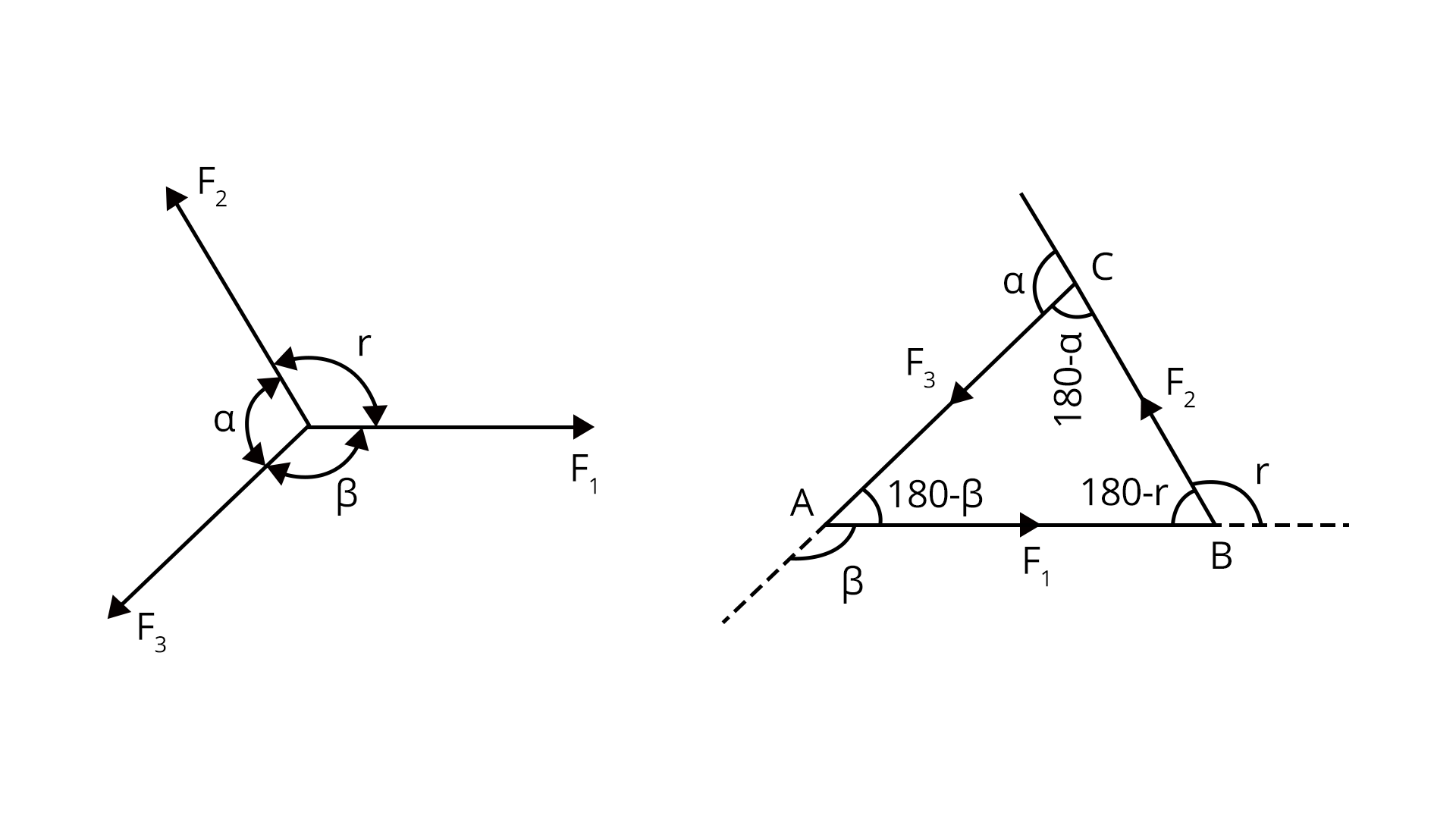Momentum and Impulse $\vec {P}= m v$$I=\int_{t_1}^{t_2}\vec {F} dt$ Law of conservation of momentum m1v1=m2v2 Miscellaneous formulae Bullets travelling with a velocity v hitting the wall:${F_{wall}}=n m v$n- Number of bulletsLiquid jet of area A moving with velocity v hits the wall:${F_{wall}}= 2 A {v^2} \cos \theta$ 7. Friction ${F_r}=\mu N$Kinetic friction Fk=k N ${F_r}={\mu_{k}} N$ 8. Centripetal Force $F= m {\omega^2}r$ 9. The apparent weight of the body in the lift (When lift moving in an upward direction) W= m (g+a)

## How Newton’s Second Law Statement Different from First Law?

Newton's First Law and Newton's 2nd Law of motion are two fundamental principles of classical mechanics, and they describe the motion of objects under the influence of forces. Here's a brief comparison between the two laws:

### Newton's First Law (Law of Inertia):

Statement: An object at rest will remain at rest, and an object in motion will remain in motion with a constant velocity unless acted upon by a net external force.

In Essence: Objects tend to maintain their state of motion (either at rest or moving with a constant velocity) unless an external force is applied.

### Newton's Second Law:

Statement: The acceleration of an object is directly proportional to the net force acting upon the object and inversely proportional to the object's mass. The direction of the acceleration is in the direction of the net force.

Mathematically: F = ma, where F is the net force, m is the mass of the object, and a is the acceleration produced.

## Differences:

### Focus:

• First Law: Deals with the absence of forces and the preservation of motion.

• Second Law: Describes the relationship between force, mass, and acceleration when forces are present.

### Inertia:

• First Law: Introduces the concept of inertia, stating that objects resist changes in motion.

• Second Law: Specifies how the motion changes when a force is applied, relating it to acceleration.

### Equations:

• First Law: No specific mathematical equation; it's more conceptual.

• Second Law: F = ma, a precise mathematical relationship among force, mass, and acceleration.

### JEE Main Laws of Motion Solved Examples

1. An object of mass 0.2 kg is thrown vertically upwards. Calculate the magnitude of the net force acting on the body and direction,

1. During its upward motion

2. During its downward motion

3. At the highest point at which the object is considered to be at rest. Will your answers change if the object was thrown at an angle of 45° in the horizontal direction and ignoring the air resistance?

Sol:

Given,

Mass of the object=m=0.2 kg

Assume value of acceleration due to gravity=g=10 m/s2

1. During upward direction

When the object is thrown upward, the acceleration due to gravity g is acting downward, therefore, the net force is acting in a downward direction.

${F_{net}}=m g= 0.2\times 10= 2N$

1. During downward motion also the net force and direction remain the same i.e, F= 2N and the direction of force acting is downwards

2. The object will not be at rest when it reaches the highest point but it will have the horizontal component of velocity. Therefore, the direction and magnitude of the net force on the object will not change even if it is thrown at 45°.

Key point: Here only acceleration that acts will be acceleration due to gravity since the object was subjected to free fall.

2. A body of mass 10 kg is acted upon by two perpendicular forces 10 N and 12 N. Calculate the magnitude and direction of the acceleration of the body.

Sol:

Given,

The mass of the object=m=10 kg

Forces acting on the body F1= 10 N and F2=12 N

Now we are asked to determine the magnitude and direction of the body. As a first step, we must calculate the resultant force acting on the body.

$\vec {F_{net}}=\sqrt{{{F_1}^2}+{{F_2}^2}+2 {F_1}{F_2} \cos \theta (\theta=90^o)}$

$\vec {F_{net}}=\sqrt{{10^2}+{12^2}}=15.62\simeq17$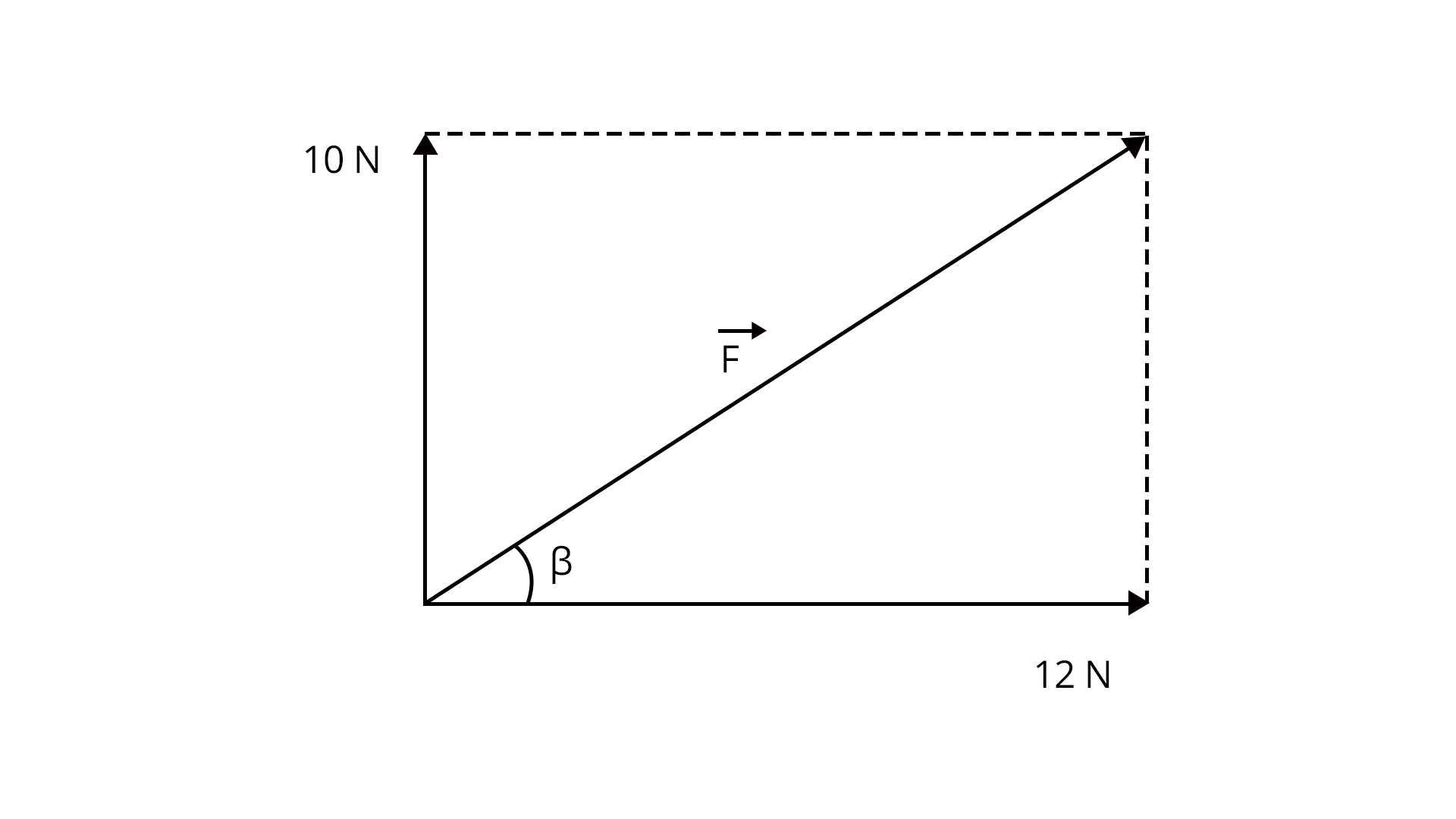The acceleration of the body,

$\vec {a}=\dfrac {\vec {F_{net}}}{m}=\dfrac {17}{10}=1.7 m/{s^2}$ in the same direction as the resultant force.

The direction of the acceleration is

$\tan \beta =\dfrac {10}{12}=0.8333$

$\beta =\tan^{-1}(0.833)= 39.7^o$ With 12 N force.

Key point: Formula for resultant force when two forces acting perpendicular to each other is $\vec {F_{net}}=\sqrt{{{F_1}^2}+{{F_2}^2}}$.

### Previous Year Questions from JEE Main Paper

1. A block of mass slides along a floor while a force of magnitude F is applied to it at an angle $\theta$ as shown in the figure. The coefficient of kinetic friction is ${\mu_k}$. Then, the block's acceleration 'a' is given by : (g is the acceleration due to gravity) (March -2021)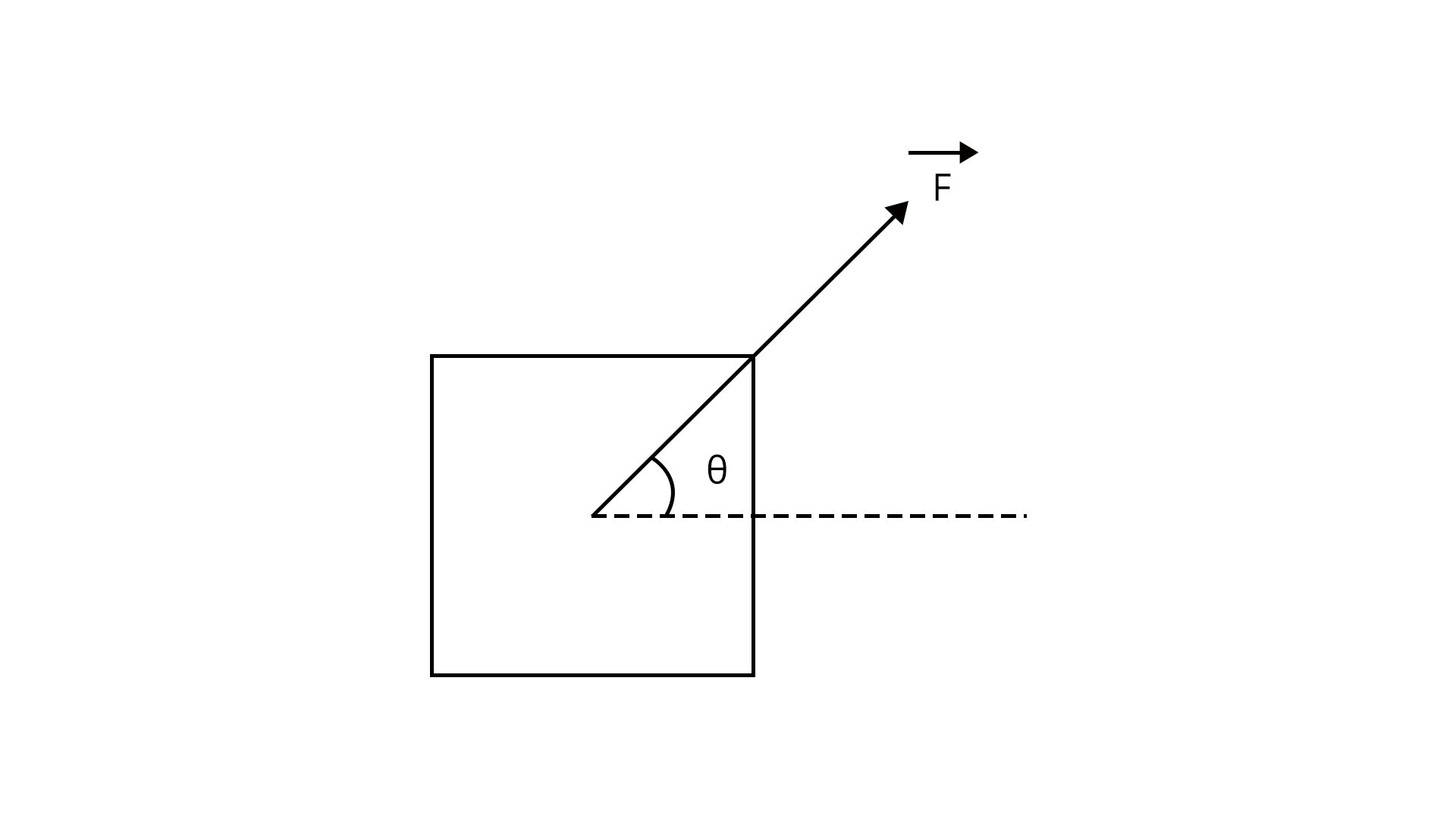Sol:

Given,

A block of mass ‘m’ is moving with the force F. We are asked to determine the acceleration of the block. This can be easily solved with the help of FBD. Let us draw the FBD for the object.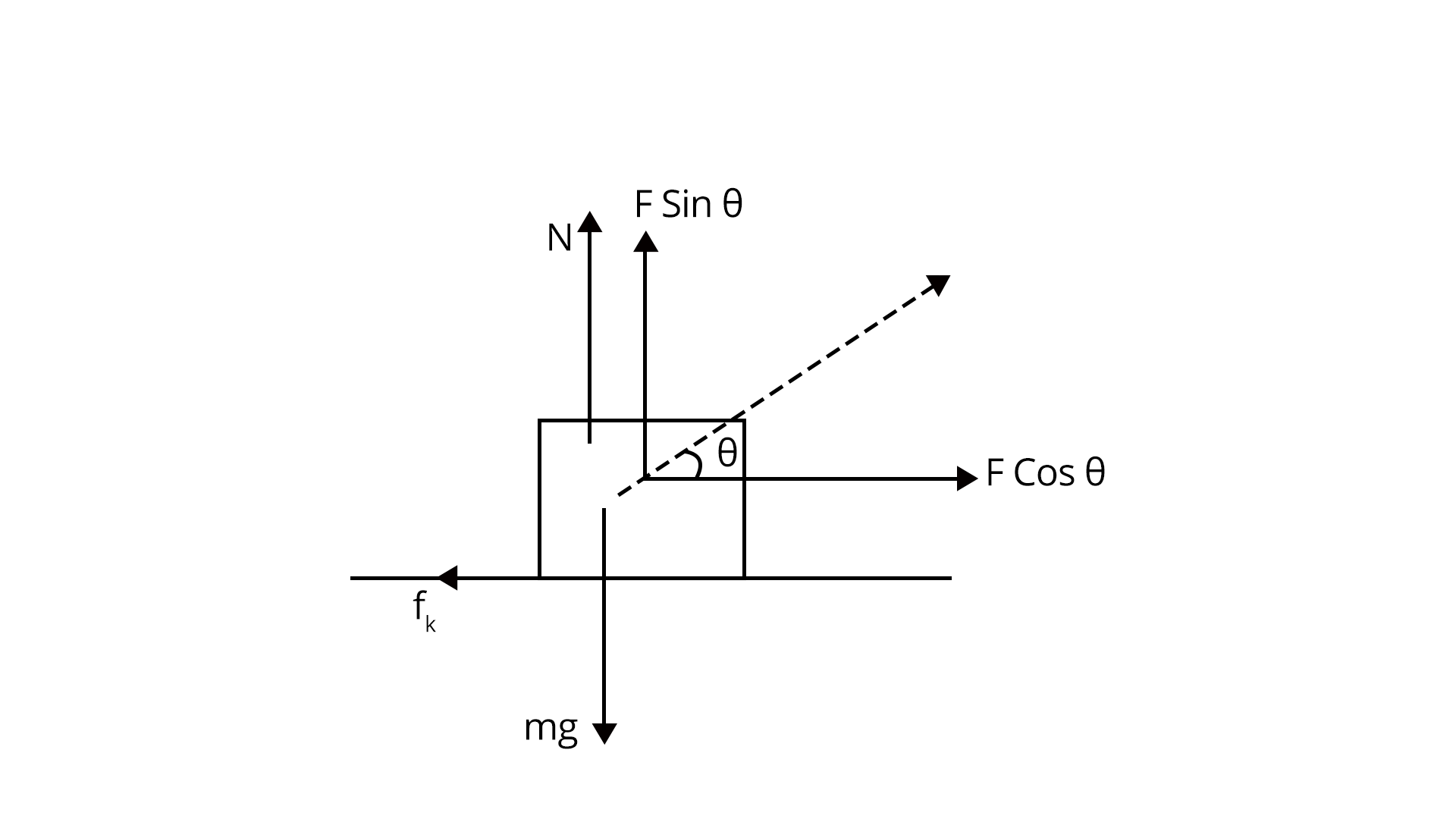From FBD we write:

$N+F \sin \theta=mg$

$\Rightarrow N=mg-F \sin \theta$

$F\cos \theta-{f_k}=ma$

$\Rightarrow F\cos \theta-{\mu_k}N=ma$

$\Rightarrow F\cos \theta-{\mu_k}(mg-F \sin \theta)=ma$

$\Rightarrow a=\dfrac {F}{m} \cos \theta -{\mu_k}(g-\dfrac {F}{m} \sin \theta)$

Trick: We can solve these problems just with the help of FBD, FBD should have all the forces acting on the body.

2. A block of mass 5 kg is (i) pushed in case (A) and (ii) pulled in case (B), by force F=20 N, making an angle of 300 with the horizontal, as shown in the figures. The coefficient of friction between the floor is $\mu = 0.2$. The difference between the accelerations of the block in case (B) and case (A) will be: (JEE-2019)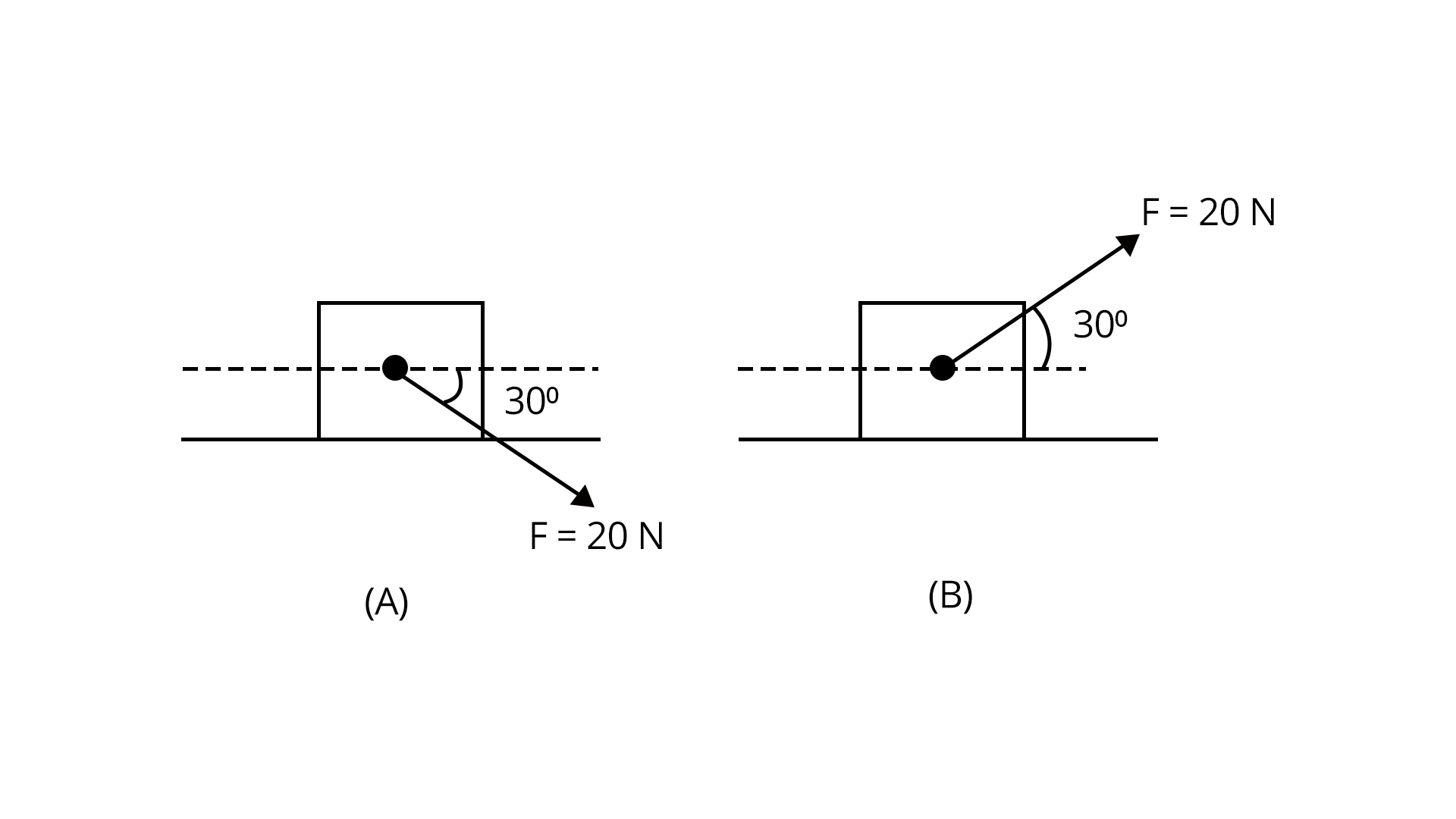Sol:

Given,

Mass of the block=m=5 kg

The magnitude of the force acting on the block=F=20 N

Case A:

In case A, the block is pushed over the surface.

Free body diagram of the block is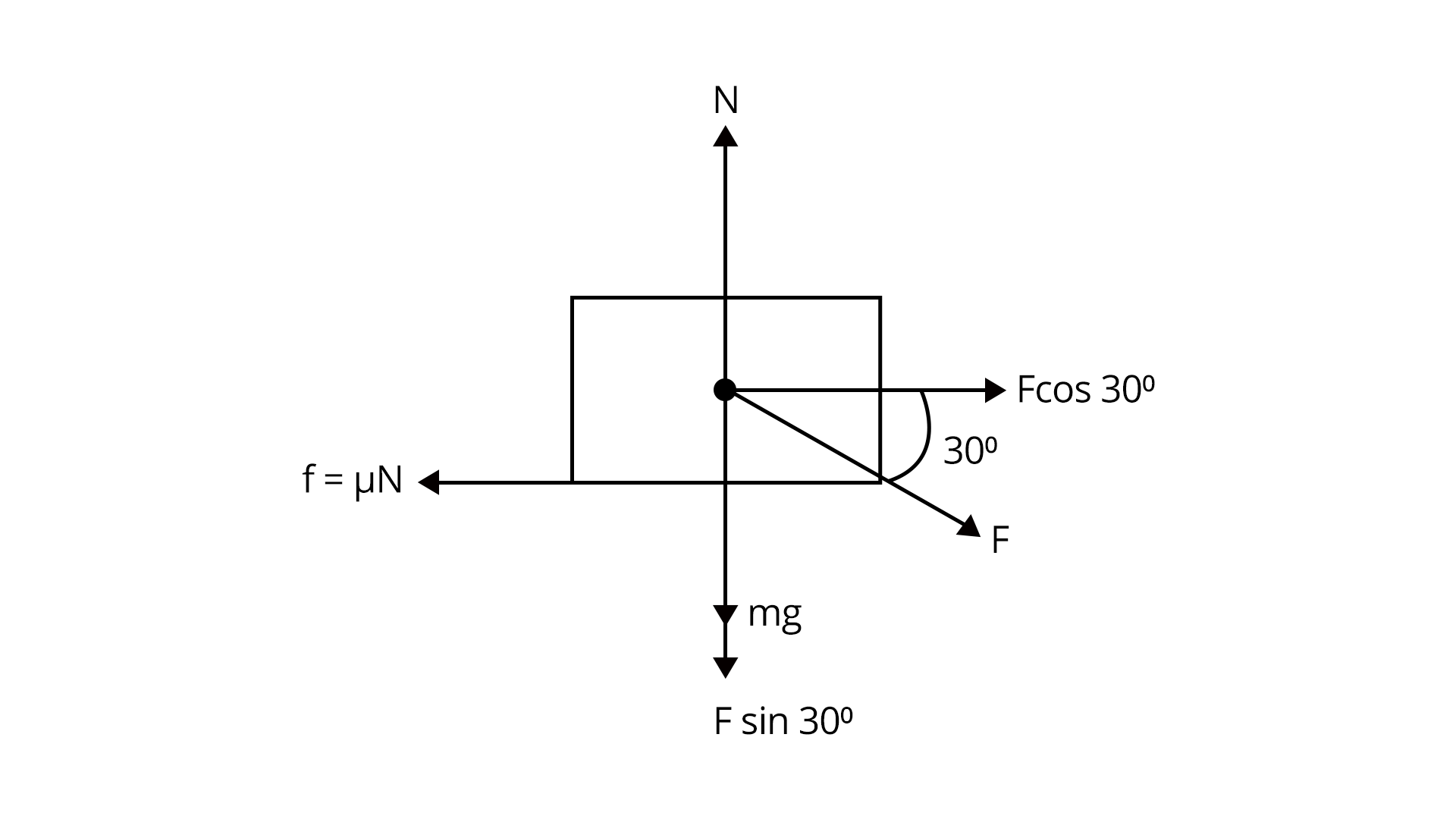From FBD we get,

${N_1}=mg+F \cos {30^o}$

${N_1}=50+ 20 (\dfrac {1}{2})=60 N$

Then the net acceleration in case A

$m {a_1}= F \cos {30^o}-{f}=20 \dfrac {\sqrt {3}}{2}-(0.2\times 60)=10 \sqrt{3}-12$

${a_1}=\dfrac {10 \sqrt{3}-12}{5}$

Case B: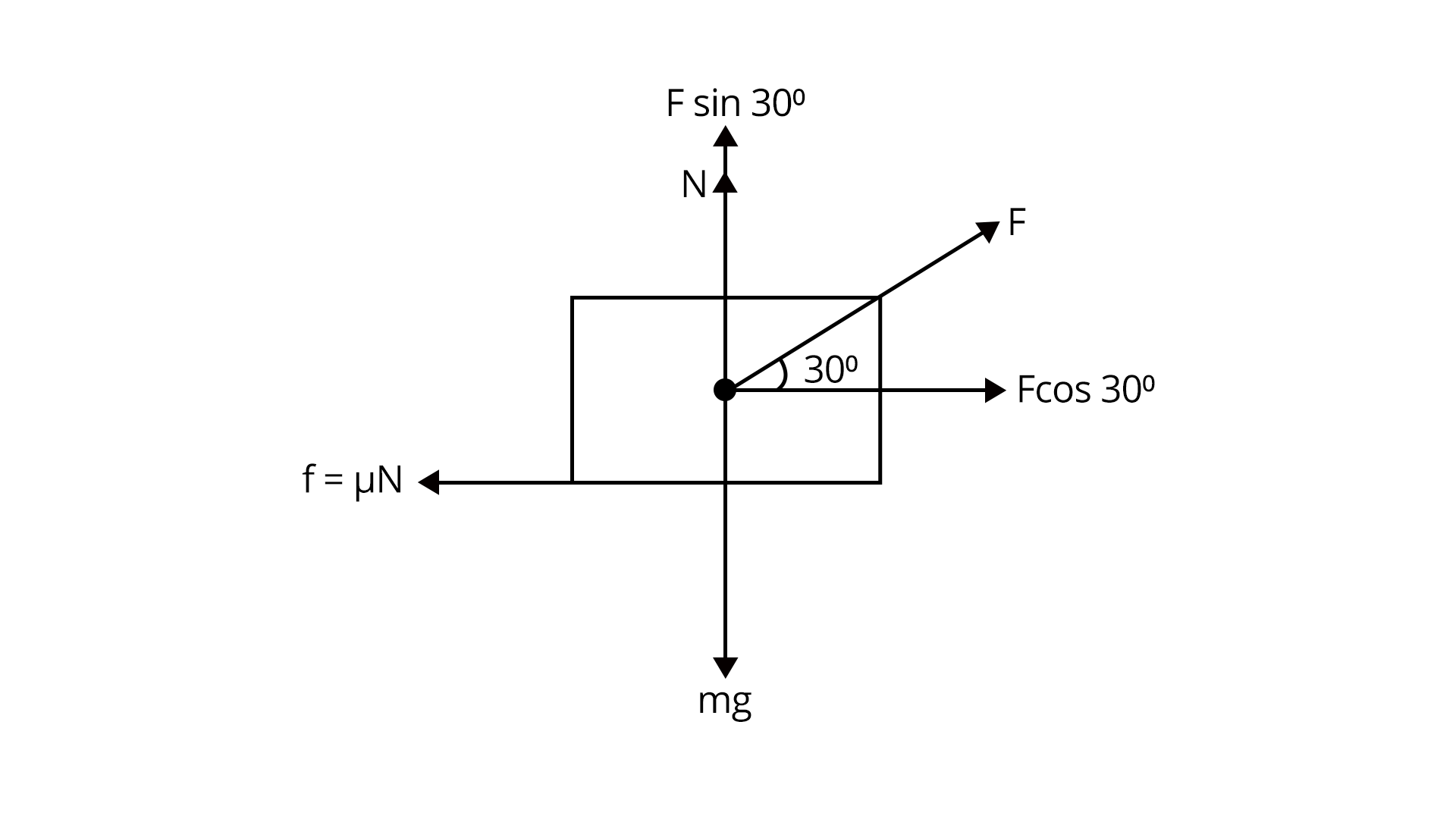From FBD, net force

Here,

$N+F \sin {30^o}=mg$

$N=mg-F \sin {30^o}$

${F_{net}}=F \cos {30^o}-{f}=10 \sqrt {3}-\mu N$

${F_{net}}=10 \sqrt {3}-\mu (mg-F \sin (30^o))=10\sqrt{3}-8$

$m{a_2}=10\sqrt{3}-8$

${a_2}=\dfrac {10\sqrt{3}-8}{5}$

Therefore,

${a_2}-{a_1}=0.8 m/{s^2}$

Trick: Resolving the given vector into components is important in order to solve the above example.

### Practice Questions

1. A block of mass 2kg rests on a rough inclined plane making an angle of 30° with the horizontal. The coefficient of static friction between the block and the plane is 0.7. The frictional force on the block is (Ans: 9.8 N)

2. A car is moving on a circular horizontal track of a radius of 10m with a constant speed of 10 m/s. A plumb bob is suspended from the roof of the car by a light rigid rod. The angle made by the rod with the track is (g = 10 m/s2)  (Ans: 450)

## JEE Main Physics Laws of Motion Study Materials

Here, you'll find a comprehensive collection of study resources for Laws of Motion designed to help you excel in your JEE Main preparation. These materials cover various topics, providing you with a range of valuable content to support your studies. Simply click on the links below to access the study materials of Laws of Motion and enhance your preparation for this challenging exam.

 JEE Main Laws of Motion Study Materials JEE Main Laws of Motion Notes JEE Main Laws of Motion Important Questions JEE Main Laws of Motion Practice Paper

## JEE Main Physics Study and Practice Materials

Explore an array of resources in the JEE Main Physics Study and Practice Materials section. Our practice materials offer a wide variety of questions, comprehensive solutions, and a realistic test experience to elevate your preparation for the JEE Main exam. These tools are indispensable for self-assessment, boosting confidence, and refining problem-solving abilities, guaranteeing your readiness for the test. Explore the links below to enrich your Physics preparation.

 JEE Main Physics Study and Practice Materials JEE  Main Physics Previous Year Question Papers JEE Main Physics Mock Test JEE Main Physics Formula JEE Main Sample Paper JEE Main Physics Difference Between

### Conclusion

In this article, we're diving into the Laws of Motion, a key chapter in JEE Main physics. We'll break down the fundamental principles and provide clear solutions to common questions. You'll grasp the core ideas, like Newton's three laws, and find practical problem-solving tips. Everything you need is right here, and you can easily access free PDFs for in-depth learning. By understanding these concepts, you'll boost your confidence for the exam, making it a valuable resource for JEE Main aspirants.

## FAQs on Laws of Motion Chapter - Physics JEE Main

1. What is the weightage of the laws of motion in JEE?

Nearly 3-4 questions arise in the exam from this chapter covering about 10 marks which makes about 4% of the total marks.

2. What are the key points that need to be practised for solving questions from laws of motion?

Students should practice how to draw the free body diagram for solving the questions from the laws of motion.

3. Are previous year's questions enough for JEE Main?

To score 200+ in MAIN, NCERT (both 11th and 12th) and previous year JEE Main papers are sufficient. Solving the previous 10-year IIT-JEE examinations offers us a tremendous advantage because 3 to 4 questions with identical alternatives are guaranteed to be repeated every year.

4. Give an example of first law of motion class 9 which can be beneficial for JEE Main Foundation Courses.

Here's an example of the first law of motion (Law of Inertia) that is beneficial for JEE Main Foundation Courses

Example: Inertial Reference Frame and Newton's First

Consider a car moving on a straight road with a constant speed. According to Newton's First Law of Motion, an object at rest will remain at rest, and an object in motion will continue to move with a constant velocity unless acted upon by a net external force.

In this scenario, the car provides an excellent illustration. When the car is moving at a constant speed on a straight road with no acceleration or deceleration, it is in an inertial reference frame. According to the first law, the car will continue to move at this constant speed unless an external force, such as braking or acceleration, is applied.

Understanding the concept of inertial reference frames and the first law of motion is fundamental to many physics problems encountered in JEE Main Foundation Courses. It lays the groundwork for more advanced topics in mechanics and helps students build a strong foundation in classical physics.

5. What is newton's first law class 9 and how it is helpful for JEE Main?

Newton's First Law: An object at rest stays at rest, and an object in motion stays in motion with the same speed and in the same direction unless acted upon by an unbalanced external force.

Understanding Newton's first law of motion class 9 is crucial for JEE Main preparation as it forms the basis of classical mechanics. Here's how it's helpful:

• Foundation Building: It establishes the concept of inertia, providing a foundation for more complex laws and principles in mechanics.

• Problem Solving: Many JEE Main problems involve analyzing forces and motion. Newton's First Law helps in identifying situations where objects remain at rest or move at a constant velocity.

• Free-Body Diagrams: Students often encounter questions requiring the construction of free-body diagrams. Newton's First Law aids in identifying the forces acting on an object.

• Application in Various Scenarios: The law is applicable to a wide range of scenarios, from projectiles to circular motion. Understanding it is key to solving diverse physics problems in the exam.

6. What is the weightage for Newton's first law class 11 in JEE Main?

The weightage for Newton's First Law of Motion (Class 11) in JEE Main can vary from year to year, and the Joint Entrance Examination (JEE) syllabus encompasses a broad range of physics topics. However, Newton's Laws of Motion, including the first law, are fundamental concepts in classical mechanics, and they are likely to have a consistent presence in JEE Main.

Typically, questions related to Newton's First Law may appear in both the theoretical and problem-solving sections of the Physics paper. It is advisable for JEE Main aspirants to thoroughly understand and practice problems related to Newton's Laws of Motion, as these principles are foundational and often serve as a basis for more complex topics in physics.

### Repeaters Course for JEE 2022 - 23## Notice board

JEE News
JEE Blogs